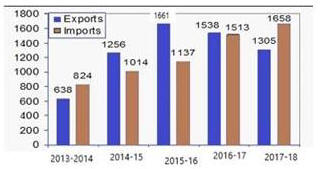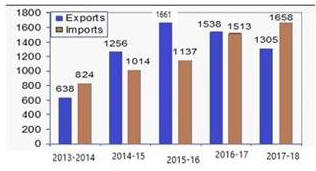# 05 July 2019 – Evening-Shift (Quantitative Aptitude)

SSC CHSL (Tier – 1) Online Exam Paper – 2018 “held on 05 JULY 2019”

Evening-Shift (Quantitative Aptitude)

Q1. Two students, A and B, appeared for an examination. A secured 8 marks more than B and the marks of the former was 55%  of the sum of their marks. the marks obtained by A and B, respectively, are:

Options

1. 36, 28
2. 44, 36
3. 38, 30
4. 40, 32

Q2. If (x + 7)³ + (2x + 8)³ +(2x + 3)³ = 3(x + 7) + (2x + 8) +(2x + 3), then what is the value of x?

Options

1. -2.4
2. 2.4
3. 3.6
4. -3.6

Q3. The ratio of the areas of two triangles ABC and PQR is 4 : 5 and the ration of their heights is 5 : 3. The ratio of the bases of triangles ABC to that of triangle PQR is :

Options

1. 11 : 15
2. 15 : 11
3. 12 : 25
4. 25 : 12

Q4. In triangle ABC, the length of BC is less than twice the length of AB by 3 cm. The length of AC exceeds the length of AB by 9 cm. The perimeter of triangle is 34 cm. The length (in cm) of the smallest side of the triangle is:

Options

1. 7
2. 8
3. 9
4. 10

Q5. The bar diagram given (bar graph) shows the import and manufacture (in tonnes) of a material manufactured by a company during the five financial years, 2013-2014 to 2017-2018.Represents the import and export (in tonnes) of a material.

Options

1. 2015-2016
2. 2016-2017
3. 2013-2014
4. 2014-2015

Q6. The simplified value of  (16/5 + 3/5) ÷ 8/5 / 8/7 ÷ {6/7 – (1/7 ÷ 1/5)} is:

Options

1. 19/16
2. 19/8
3. 19/7
4. 19/64

Q7. If a 10-digit number 1230x558y2 is divisible by 88, then the value of (5x + 5y) is:

Options

1. 50
2. 40
3. 20
4. 30

Q8. A, B and  C can complete a piece of work in 4,20 and 60 days respectively. Working together, they can complete the one third of the same work in how many days?

Options

1. 20/19
2. 22/19
3. 3/19
4. 39/19

Q9. If 3 ³√x + 4 ³√x = 5³√x then the value of x is:

Options

1. 8
2. 2
3. 4
4. 1

Q10. If sec = 3 x and tan = 3/x , (x 0) then the value of 9(x² – 1/x²) is:

Options

1. 1
2. 1/4
3. 1/3
4. 1/2

Q11. There was 25% off on shirt. A lady bought a shirt and got an additional 20% discount for paying in cash and further 10% discount for being a loyal customer. She paid Rs.405. What was the tag (in Rs) on shirt?

Options

1. 750
2. 725
3. 600
4. 650

Q12. If x% is 150 and y% of Z is 300, then the relation between x and z is:

Options

1. z=x/2
2.z = x
3.z = 2x
4.z = x/3

Q13. If x = 2-√3 then the value of x³ – x-3 is:

Options

1. -30√2
2.-30√3
3. 30√2
4. 30√2

Q14. Two numbers are in the ration of 7:5. On diminishing each of them by 40, the ratio becomes 27:17. The sum of the numbers is:

Options

1. 275
2. 300
3. 240
4. 325

Q15. A person purchased a vehicle for Rs 4,90,828 and sold it for Rs 5,52,920. What is the percent profit earned on the vehicle (correct to two decimal places) ?

Options

1. 19.55 %
2. 15.51 %
3. 11.55 %
4. 12.65 %

Q16. The Bar Graph presents the Import and Exports of an item (in tonnes) manufactured by a company for the five financial years, 2013-2014 to 2017-2018.What is the average of absolute difference between Exports and Imports (in tonnes) the five financial years?

Options

1. 266
2. 264
3. 260
4. 256

Q17.  In a ∠ABC, the bisectors of ∠B and ∠C meet at point O within the triangle. If ∠A = 132°, then the measure of BOC is:

Options

1. 132°
2. 84°
3. 156°
4. 66°

Q18. If cos x = -1/2 and π < x < 3π /0, then the value of  2tan²x + 3cosec²x is:

Options

1. 16
2. 4
3. 8
4. 10

Q19. The Bar Graph presents the Import and Exports of an item (in tonnes) manufactured by a company for the five financial years, 2013-2014 to 2017-2018.In which financial year the percentage increase in Imports and Exports taken together is the lowest in comparison to its previous financial year?

Options

1. 2015-2016
2. 2014-2015
3. 2017-2018
4. 2016-2017

Q20. The Bar Graph presents the Import and Exports of an item (in tonnes) manufactured by a company for the five financial years, 2013-2014 to 2017-2018.What is the ratio of total Imports to total Exports during 2014-2015 and 2016-2017?

Options

1. 3664 : 4455
2. 4175 : 4011
3. 4445 : 3664
4. 4011 : 4175

Q21. The ratio between the speed of two trains is 5 : 7. If the first train covers 300 km in 3 hours, then the speed (in km/h) of the first train is:

Options

1. 150
2. 100
3. 140
4. 120

Q22. The average age of four brothers is 14 years. If their father is also included, the average is increased by 4 years. The age of the father (in years) is:

Options

1. 34
2. 36
3. 38
4. 40

Q23. The chord of the contact of tangents drawn from a point on the circle x² + y² = a² to the circle x² + y² = b² touches the circle x² + y² = c² such that , where m,n,p∈N, and m,n,p are prime to each other, then the value of m + n + p + 3 is:

Options

1. 5
2. 6
3. 2
4. 7

Q24. A circle is inscribed in  a triangle ABC. If touches sides AB,BC and AC at the points P, Q and R respectively. If BP = 5.4 cm, CQ = 7.3 cm and AR = 6.1 cm, then the perimeter (in cm) of the∠ABC is:

Options

1. 37.6
2. 37.25
3. 36
4. 37